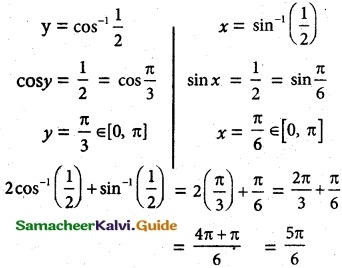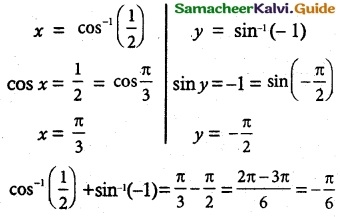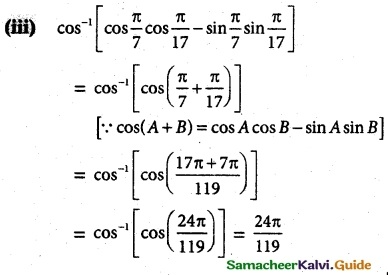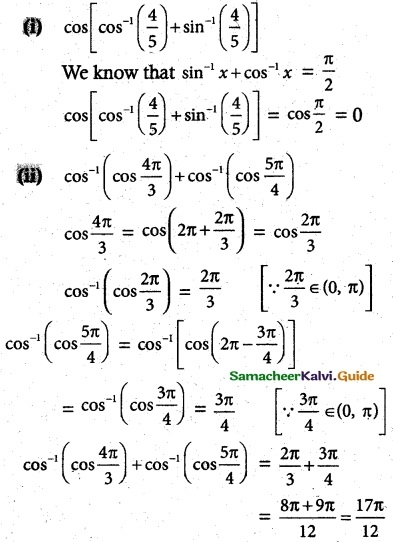Tamilnadu State Board New Syllabus Samacheer Kalvi 12th Maths Guide Pdf Chapter 4 Inverse Trigonometric Functions Ex 4.2 Textbook Questions and Answers, Notes.

## Tamilnadu Samacheer Kalvi 12th Maths Solutions Chapter 4 Inverse Trigonometric Functions Ex 4.2

Question 1.
Find all values of x such that
(j) -6π ≤ x ≤ 6π and cos x = 0
(ii) -5π ≤ x ≤ 5π and cos x = 1
Solution:
(i) cos x = 0
cos x = cos $$\frac {π}{2}$$
x = (2n + 1) $$\frac {π}{2}$$, n = 0, ±1, ±2, ±3, ±4, ±5, -6

(ii) cos x = -1
cos x = cos π
x = (2n + 1) π, n = 0, ±1, ±2, -3Question 2.
state the reason for cos -1 [cos($$\frac {-π}{6}$$)] ≠ –$$\frac {π}{6}$$
Solution:
cos -1 [cos(-$$\frac {π}{6}$$)] = cos -1[ $$\frac {π}{6}$$ ] = $$\frac {π}{6}$$ ≠ $$\frac {-π}{6}$$ ∉ [0, π]
Which is the principle domain of cosine function [∵ cos(-θ) = cos θ]

Question 3.
solution:Question 4.
Find the principle value of cos-1($$\frac {1}{2}$$)
solution:
y = cos-1($$\frac {1}{2}$$)
cos-1x range is [0, π]
cos y = $$\frac {1}{2}$$ = cos = $$\frac {π}{3}$$
y = $$\frac {π}{3}$$ ∈ [0, π]
principle value is $$\frac {π}{3}$$

Question 5.
find the value of
(i) 2 cos-1 ($$\frac {1}{2}$$) + sin-1 ($$\frac {1}{2}$$)
(ii) cos-1 ($$\frac {1}{2}$$) + sin-1(-1)
(iii) cos-1 (cos$$\frac {π}{2}$$cos$$\frac {π}{17}$$ – sin$$\frac {π}{7}$$sin$$\frac {π}{17}$$)
Solution:
(i) 2 cos-1 $$\frac {1}{2}$$ + sin-1 $$\frac {1}{2}$$(ii) cos-1 $$\frac {1}{2}$$ + sin-1(-1)Question 6.
Find the domain of
(i) f(x) = sin-1 ($$\frac {|x|-2}{3}$$) + cos-1 ($$\frac {1-|x|}{4}$$)
(ii) g(x) = sin-1 x + cos-1 x
Solution:
(i) -1 ≤ sin-1 (x) ≤ 1
-1 ≤ $$\frac {|x|-2}{3}$$ ≤ 1
-3 ≤ |x| – 2 ≤ 3
-3 + 2 ≤ |x| ≤ 3 + 2
-1 ≤ |x| ≤ 5
|x| ≤ 5
since -1 ≤ |x| is not possible
-5 ≤ x ≤ 5 ………. (1)
By the definitions
-1 ≤ cos-1 (x) ≤ 1
-1 ≤ $$\frac {1-|x|}{4}$$ ≤ 1
-4 ≤ 1 – |x| ≤ 4
-5 ≤ -|x| ≤ 3
-3 ≤ |x| ≤ 5
-3 ≤ |x| is not possible
-5 ≤ x ≤ 5 ………. (2)
From 1 and 2 we get
domain is x ∈ [-5, 5](ii) g(x) = sin-1 x + cos-1 x
range of sin x and cos x is [-1, 1]
-1 ≤ x ≤ 1
∴ x ∈ [-1, 1]
The domain of g(x) = [-1, 1]

Question 7.
For what value of x, the inequality
$$\frac {π}{2}$$ < cos-1 (3x – 1) < π holds?
Solution:
$$\frac {π}{2}$$ < cos-1 (3x – 1) < π
cos $$\frac {π}{2}$$ < (3x – 1) < cos π
0 < 3x < 1 < -1
0 + 1 < 3x < -1 + 1
1 < 3x < 0
The inequality is true, only when
0 < x < $$\frac {1}{3}$$Question 8.
Find the value of
(i) cos[cos-1($$\frac {4}{5}$$) + sin-1($$\frac {4}{5}$$)]
(ii) (cos-1(cos $$\frac {4π}{3}$$)) + cos-1(cos($$\frac {5π}{4}$$))
Solution: